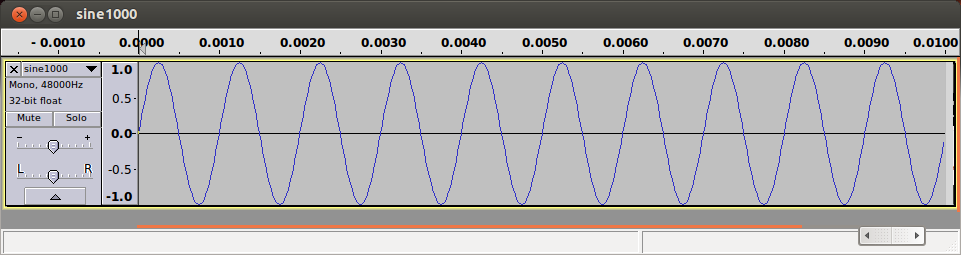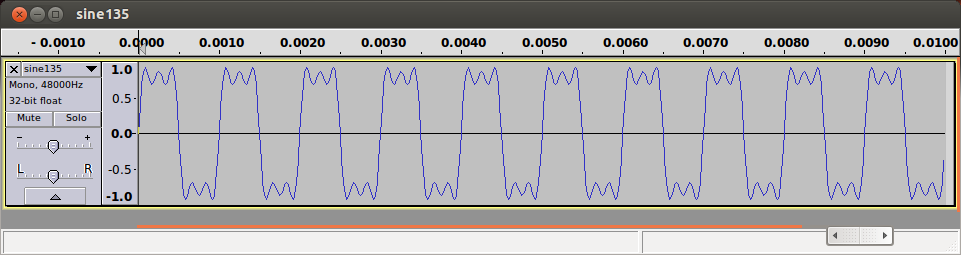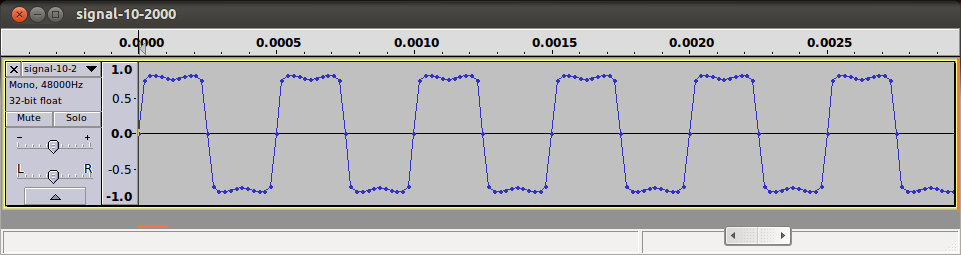# Using sox and Audacity to Demonstrate Communication Signals

In the Introduction to Data Communications course I teach we cover basics of how communication signals are composed of sinusoid signals (c.f. Fourier transforms). Its nice to be able to demonstrate how different sinusoids can be combined. Although I have examples in my slides, generating signals in class is also interesting. In the past I've used Matlab and Scilab to create signal plots. This year I tried using sox to generate simple signals, and then Audacity to view the signals (including frequency spectrum). Below are some basic steps I used.

Generate a 1000 Hz sine wave, s1(t) with duration of 0.01 seconds, saving the output in `sine1000.wav`:

s1(t) = sin(2000 π t)

``````\$ sox --null sine1000.wav synth 0.01 sine 1000
``````

A plot of the signal in Audacity:Similar can be applied to create sine waves of different frequencies, e.g. 3000 Hz and 5000 Hz.

To add the sine waves together they can be mixed with sox. The amplitude of each component can be set using the --volume option when mixing:

s(t) = sin(2000 π t) + (1/3) sin(6000 π t) + (1/5) sin(10000 π t)

``````\$ sox --combine mix sine1000.wav --volume 0.333 sine3000.wav --volume 0.2 sine5000.wav sine135.wav
``````To automate the process of creating signals by combining multiple sinusoid components I created a simple Bash script called generate-sine-signal.

``````#!/bin/bash
# Generate sine waves and then combine them to produce signal
# Uses sox as easy to use on command line. Optionally audacity can
# then be used to look at plots of the signals including frequency
# spectrum.
# Demonstrates basic concept of combining sinusoids to create
# digital sqare wave
# Usage:
# generate-sine-signal components fundamental duration
#   components: integer > 1 indicating number of sine components
#   fundamental: fundamental frequency in Hz
#   duration: duration of signal in seconds
# Steven Gordon

# Input parameters
components=\$1
fundamental=\$2
duration=\$3

# Start of names for output files
sine="sine"
signal="signal"

# Precision for calculations
bcscale=5

# Command to combine the sine waves
soxsignal="sox --combine mix"
extraname=""

# For each component generate a sine wave and add to list to combine
for i in `seq 1 \${components}`
do
# n, i.e. 1, 3, 5, ...
n=`echo "scale=\${bcscale}; \${i} * 2 - 1" | bc `

# Frequency of the component
frequency=`echo "scale=\${bcscale}; \${n} * \${fundamental}" | bc`

# Amplitude of the component
a=`echo "scale=\${bcscale}; 1 / \${n}" | bc`

# Generate the sine wave with sox
sox --null \${sine}-\${frequency}.wav synth \${duration} sine \${frequency}

# Mix with other sine waves
soxsignal="\${soxsignal} --volume \${a} \${sine}-\${frequency}.wav"
extraname="\${extraname}-\${frequency}"
done

# Generate the signal with sox
\${soxsignal} \${signal}-\${components}-\${fundamental}.wav
``````

For example, to generate a signal with 10 components, fundamental frequency of 2000 Hz and duration of 3 seconds:

``````\$ generate-sine-signal 10 2000 3
``````

The resulting file (signal-10-2000.wav) plotted in Audacity: